# 14. 机器学习 - KNN & 贝叶斯Hi，你好。我是茶桁。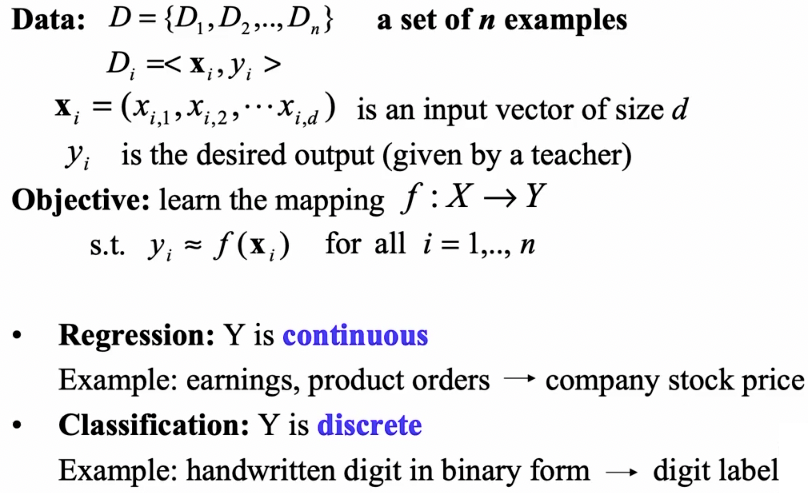## KNN

KNN 几乎可以说是最简单、最直接、最古老的一种机器学习方法了。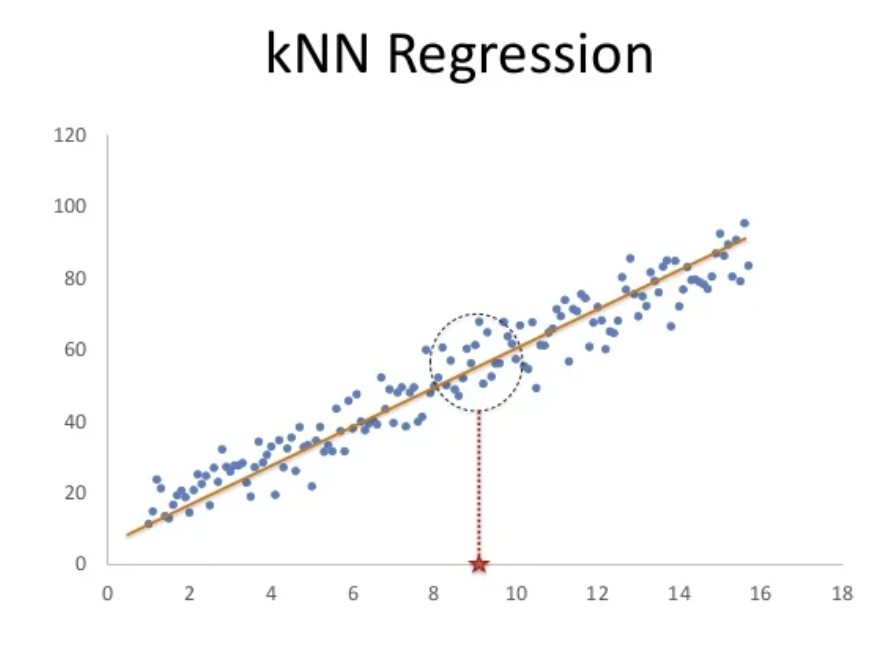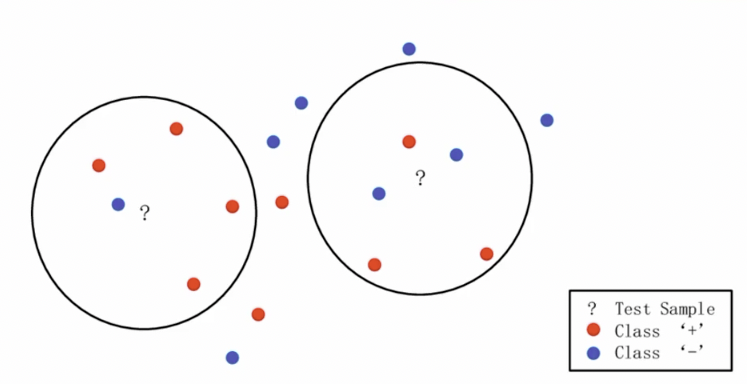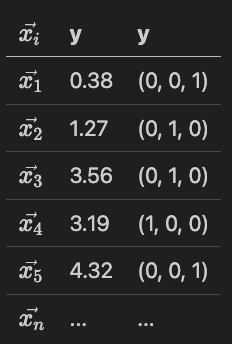KNN 找的是和自己最类似的，但是如果他找不到和自己最类似的，他就傻了。

2.7Ghz，Ghz 就是一秒钟可以运行一个 G，一个 G 就是 2 的 11 次方。

lazy-learning 就是懒惰的学习，KNN 就是这种方式。KNN 是典型的一种 lazy-learning。

KNN 只是简单的内容记下来，然后去找了一个最接近的东西。lazy-learning 最大的问题就是所观测到的是它周围的这些结果。

Data site 是比较少的维度，其实效果倒也可以。

## 贝叶斯

Pr(购买 | 本科及以上) = Pr(本科及以上 | 购买) * Pr(购买) / Pr(本科及以上) = 15% * 7% / 5% = 21%

Pr(购买 | 本科以下) = Pr(本科以下 | 购买) * Pr(购买) / Pr(本科以下) = 85% * 7% / 95% = 6.26%

\begin{align*} P(A|B) = \frac{P(B|A)*P(A)}{P(B)} \end{align*}

• P(A|B) 是后验概率，表示在给定观测数据 B 后事件 A 发生的概率。
• P(B|A) 是条件概率，表示事件 A 发生的情况下事件 B 发生的概率。
• P(A) 是先验概率，表示事件 A 在没有观测数据 B 的情况下的概率。
• P(B) 是边际概率，表示事件 B 发生的总概率。

\begin{align*} p(C_k,x_1,...,x_n) & = p(x_1,...,x_n, C_k) \\ & = p(x_1|x_2,...,x_n,C_k)p(x_2,...,x_n,C_k) \\ & = p(x_1|x_2,...,x_n,C_k)p(x_2|x_3,...,x_n,C_k)p(x_3,...,x_n,C_k) \\ & = ... \\ & = p(x_1|x_2,...,x_n,C_k)p(x_2|x_3,...,x_n,C_k)...p(x_{n-1}|x_n,C_k)p(x_n|C_k)p(C_k) \end{align*}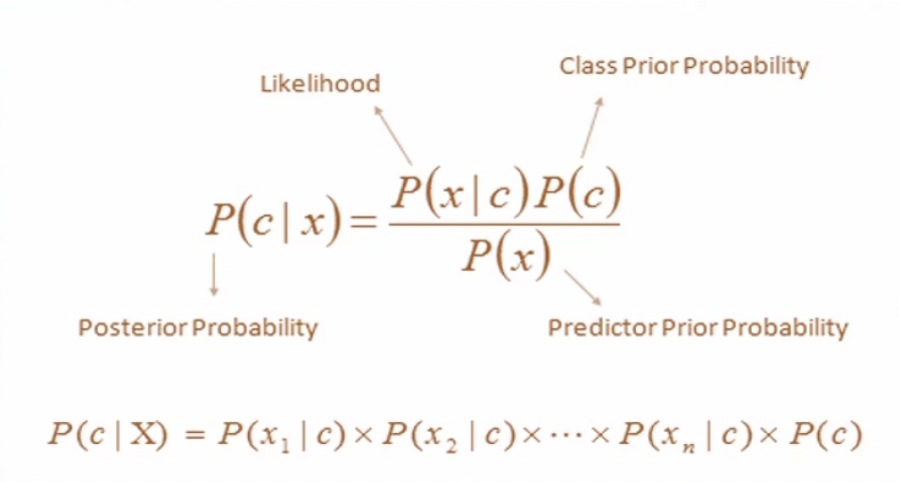• P(C|X) 是后验概率，表示在给定特征向量 X 的情况下，样本属于类别 C 的概率。
• P(X|C): 是似然度，表示在类别 C 下观测到特征向量 X 的概率。基于朴素独立性假设，可以将它分解为各个特征的条件概率的乘积：$P(X|C) = P(x_1|C)*P(x_2|C)*...*P(x_n|C)$
• P(C) 是先验概率，表示样本属于类别 C 的概率。
• P(X) 是归一化常数，用于确保后验概率的总和为 1。

\begin{align*} C_{MAP} = \arg max_cP(C|X) \end{align*}

Example No. Color Type Origin Stolen?
1 Red Sports Domestic YES
2 Red Sports Domestic NO
3 Red Sports Domestic YES
4 Yellow Sports Domestic NO
5 Yellow Sports Imported YES
6 Yellow SUV Imported NO
7 Yellow SUV Imported YES
8 Yellow SUV Domestic NO
9 Red SUV Imported NO
10 Red Sports Imported YES

\begin{align*} P(C_1 |x) = \frac{分子}{P(x)} \\ P(C_2 |x) = \frac{分子}{P(x)} \\ \end{align*}

\begin{align*} P(C_1|x) = P(x_1|C_1)P(x_2|C_1)P(x_3|C_1)P(C_1) \\ P(C_2|x) = P(x_1|C_2)P(x_2|C_2)P(x_3|C_2)P(C_2) \end{align*}

$P(C_1)$$P(C_2)$的概率是多少？$P(C_1)$$P(C_2)$是我们所有的汽车里面，被偷的占比多少，没偷的占比多少，也是数数就可以数出来。

\begin{align*} p(x = v|C_k) = \frac{1}{\sqrt{2\pi\sigma^2_k}}e^{-\frac{(v-\mu k)^2}{2\sigma^2_k}} \end{align*}

## 贝叶斯案例 - 预测广告

• Text: 今天什么时候回家

\begin{align*} Pr(Ad|w_1 w_2 w_3) = \frac{Pr(w_1|Ad)Pr(w_2|Ad)Pr(w_3|Ad)Pr(Ad)}{Pr(w_1w_2w_3)} \end{align*}

\begin{align*} Pr(Text|w_1 w_2 w_3) = \frac{Pr(w_1|Text)Pr(w_2|Text)Pr(w_3|Text)Pr(Text)}{Pr(w_1w_2w_3)} \end{align*}

\begin{align*} Pr(Ad|S) & = 1/2 * 1/13 * 2/3 = 1/13 * 1/3 \\ Pr(Text|S) & = 2/13*1/3 \end{align*}Hivan Du

2023-10-28

2023-11-25